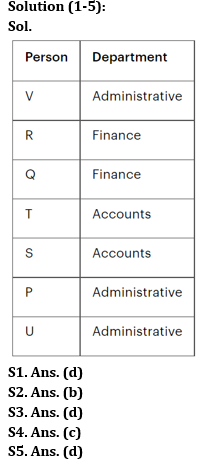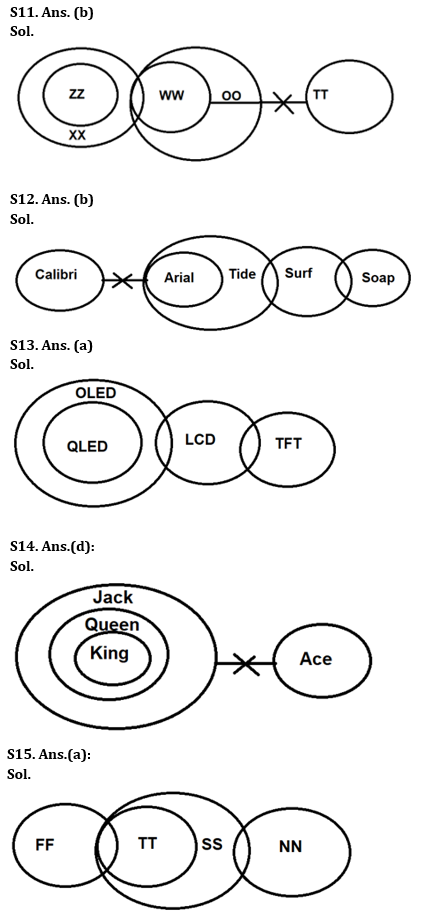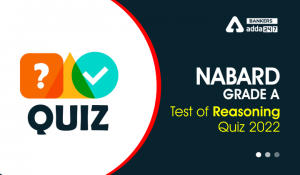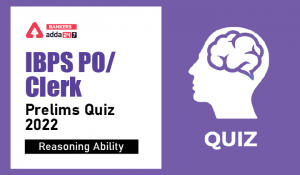Latest Banking jobs   »

# Reasoning Ability Quiz For IBPS RRB PO Prelims 2022- 5th July

Directions (1-5): Answer the following question based on the information given below:
Seven people, P, Q, R, S, T, U and V, work in three different departments (Accounts, Administrative and Finance). At least two and at most three people work in the same department.
T and S work in the same department. Only one more person is working in the same department as R. S and V do not work in the same department. P and U work in the same department. V works in administrative department. R and V do not work in the same department. Q neither works in Accounts department nor with T.

Q1. Who among the following person works in same department as R?
(a) P
(b) T
(c) S
(d) Q
(e) U

Q2. Which of the following statement is true?
(a) V and Q work in the same department
(b) S works in Account department
(c) U and P work in Finance department
(d) Q works in administrative department
(e) None of the above statement is true

Q3. P works in _________ department with_________?
(b) Accounts, S
(c) Finance, R
(e) Accounts, U

Q4. Who among the following person works in Account department?
(a) U
(b) P
(c) T
(d) R
(e) None of these

Q5. Which of the following combination is incorrect?
(c) T – Accounts
(d) R – Accounts
(e) Q – Finance

Directions (6-10): study the given arrangement of numbers, symbols & alphabets and answer the questions based on it.

1 A B 2 C D 4 ! F G H I J K L M N ^ O 6 E 5 \$ & P Q 7 8 % 9 R S T # U * V

Q6. How many even numbers are there in between the element which is 6th from left and element which is 11th from right end?
(a) One
(b) Two
(c) Three
(d) Four
(e) None of the above

Q7. If we remove all the vowels from the given arrangement then which is exactly in the middle of ! and 6 in new arrangement?
(a) J
(b) H
(c) K
(d) L
(e) None of the above

Q8. If all the symbols are dropped then which element is eighth to the right of eighth element from left end?
(a) N
(b) M
(c) O
(d) 6
(e) None of the above

Q9. How many symbols are between 2nd number from left end and 2nd number from right end?
(a) 2
(b) 3
(c) 4
(d) 5
(e) None of the above

Q10. If all the numbers are dropped then which element is 2nd to the left of 19th element from left end?
(a) M
(b) E
(c) O
(d) ^
(e) None of the above

Directions (11-15): In the question below four statements are given followed by two conclusions numbered I and II. You have to take the given statements to be true even if they seem to be at variance with commonly known facts. Read all the conclusions and then decide which of the given conclusions logically follows from the given statements disregarding commonly known facts.

Q11. Statements:
All ZZ is XX. A few XX is WW. Each WW is OO. No TT is WW.
Conclusions:
I. A few ZZ is OO
II. All XX being OO is a possibility
(a) If only conclusion I follows
(b) If only conclusion II follows
(c) If either conclusion I or conclusion II follows
(d) If both conclusions I and II follow
(e) If neither conclusion I nor conclusion II follows

Q12. Statements:
No Calibri is Arial. All Arial is Tide. Only a few Tides is Surf. Some Surf is Soap.
Conclusions:
I. A few Tides is Calibri
II. All Surf being Tide is a possibility
(a) If only conclusion I follows
(b) If only conclusion II follows
(c) If either conclusion I or conclusion II follows
(d) If both conclusions I and II follow
(e) If neither conclusion I nor conclusion II follows

Q13. Statements:
Only OLED is QLED. Some LCD is OLED. Some TFT are LCD.
Conclusions:
I. Some OLED are QLED
II. Some OLED are TFT
(a) If only conclusion I follows
(b) If only conclusion II follows
(c) If either conclusion I or conclusion II follows
(d) If both conclusions I and II follow
(e) If neither conclusion I nor conclusion II follows

Q14. Statements:
All King are Queen. Each Queen is Jack. No Ace is Jack.
Conclusions:
I. Some Jack is King
II. No Queen is Ace
(a) If only conclusion I follows
(b) If only conclusion II follows
(c) If either conclusion I or conclusion II follows
(d) If both conclusions I and II follow
(e) If neither conclusion I nor conclusion II follows

Q15. Statements:
Only a few FF is TT. All TT is SS. Only a few SS is NN.
Conclusions:
I. All NN being SS is a possibility
II. Mostly FF is NN
(a) If only conclusion I follows
(b) If only conclusion II follows
(c) If either conclusion I or conclusion II follows
(d) If both conclusions I and II follow
(e) If neither conclusion I nor conclusion II follows

SolutionsS6. Ans. (b)
Sol. Given Series – 1 A B 2 C D 4 ! F G H I J K L M N ^ O 6 E 5 \$ & P Q 7 8 % 9 R S T # U * V
Hence, there are only two even numbers (4 and 6) between the element which is 6th from left end and 11th from right end.

S7. Ans. (c)
Sol. Given Series – 1 A B 2 C D 4 ! F G H I J K L M N ^ O 6 E 5 \$ & P Q 7 8 % 9 R S T # U * V
After removing all the vowels, we get K is exactly middle element between ! and 6.

S8. Ans. (a)
Sol. Given Series – 1 A B 2 C D 4 ! F G H I J K L M N ^ O 6 E 5 \$ & P Q 7 8 % 9 R S T # U * V
After dropping symbols: 1 A B 2 C D 4 F G H I J K L M N O 6 E 5 P Q 7 8 9 R S T U V
So, N is eighth to the right of eighth element from left end.

S9. Ans. (c)
Sol. Given Series – 1 A B 2 C D 4 ! F G H I J K L M N ^ O 6 E 5 \$ & P Q 7 8 % 9 R S T # U * V
There are 4 symbols (!, ^, \$, &) between 2nd number from left end and 2nd number from right end.

S10. Ans. (b)
Sol. Given Series – 1 A B 2 C D 4 ! F G H I J K L M N ^ O 6 E 5 \$ & P Q 7 8 % 9 R S T # U * V
After dropping numbers: A B C D ! F G H I J K L M N ^ O E \$ & P Q % R S T # U * V
So, E is second to the left of 19th element from left end.#### Congratulations!Incorrect details? Fill the form again here

•Reasoning Ability Quiz For IBPS RRB PO C...
•Reasoning Ability Quiz For NABARD Grade ...
•Reasoning Ability Quiz For IBPS Clerk/PO...
•Reasoning Ability Quiz For IBPS RRB PO C...
•Reasoning Ability Quiz For IBPS RRB PO C...
•Reasoning Ability Quiz For IBPS Clerk Pr...